# 交流電路中的被動元件單擊或點擊下面的示例電路以調用TINACloud並選擇交互式DC模式以在線分析它們。

VM = R *IM or V = R *I

VM = R * IM or V = R * I.1. 對於 高峰 (VM，我M ）或 有效 當前和的（V，I）值 電壓：

VM = XL*IM or V = X.L*I

2. 使用複雜的相量：

VM = j * XL IM or V = j * XL * I

ZL= V/I = VM / IM = j w L

YL= I / V = IM /VM = 1 /（j w L)

VM = XL IM or V = X.L * 一世

 證明 純線性電壓的時間函數 感應器 通過考慮與電感器的電壓和電流相關的時間函數，可以找到（內部電阻為零且無雜散電容的電感器）：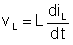. 使用上一章介紹的複雜時間函數概念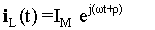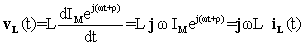使用複雜的相量： VL = j w L* IL 或具有實時功能 vL （t）= w L iL （T + 90°) 所以電壓是90° 在當前之前。

ILM= VLM/(jwL）= 1 /（j6.28 100 * * 0.001）= - j1.59A

iL（t）= 1.59sin（wT-90°） 一個。#### 例如1

XL = 2 *p* f * L = 2 * 3.14 * 50 * 0.003 = 0.9425歐姆= 942.5歐姆

ZL= j w L = j 0.9425 = 0.9425 j 歐姆#### 例如2

XL = 2 *p* f * L = 2 * 3.14 * 200 * 3 = 3769.91歐姆° 背後 （在一個 逆時針方向）的電流。 在復平面中，電壓相量在負方向（相對於參考方向，逆時針）上垂直於電流相量。 您可以使用虛數以復數來表示–j 作為乘數。

1。 為了 絕對峰值 or 有效 當前和的價值 電壓：or V = X.C*I

2。 為了 複雜的高峰 or 有效 電流和電壓值：

VM = –j * XC*IM or V = – Ĵ* XC*I

ZC = V / I = VM / IM = – j*XC = – j / wC

YC= I / V = IM / VM = j wC)

 證明： 純線性電容上的電壓的時間函數（無並聯或串聯電阻且無雜散電感的電容器） 可以用電容器電壓的時間函數表示（vC），費用（qC）和當前（iC ):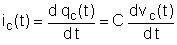如果C不依賴於時間，則使用複雜的時間函數： iC（t）= j w C vC（t）的 or vC（t）=（ - 1 /jwC）*iC（t）的 或使用複數相量：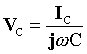或具有實時功能 vc （t）= ic （叔90°）/（w C) 所以電壓是90° 背後 目前。

ICM= jwCVCM =j6.28*10610-7 * 2）=j1.26，

iL（t）= 1.26sin（wt + 90°）一個

#### 例如3

XC = 1 /（2 *p*f*C) = 1/(2*3.14*50*25*10-6）= 127.32歐姆

Z-C= 1 /（j w C）= – j 127.32 = –127.32 j 歐姆XC = 1 /（2 *p*f*C) = 1/(2*3.14*200*103* 25 * 10-6）= 0.0318 = 31.8 mohms。Z = V / I = VM/IM

ZR = R; ZL = j w L ZC = 1 /（j w C）= –j / wC

X很高興有你 DesignSoft
如果需要任何幫助找到合適的產品或需要支持，可以進行聊天。